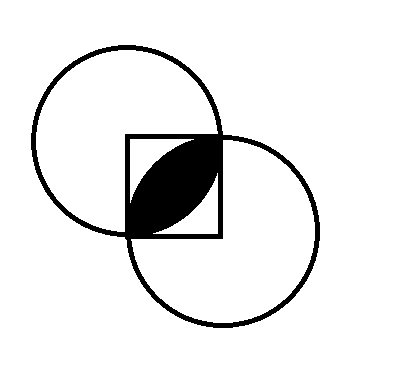# Intersecting Circles

Geometry Level 2A square with side length of 5 is drawn. Using a vertex of the square as the center and the square's side length as its radius, a circle is drawn. Using the vertex diagonally opposite of the one we just used as the center and the square's side length as its radius, another circle is drawn. Find the area of the intersection between the two circles.

×